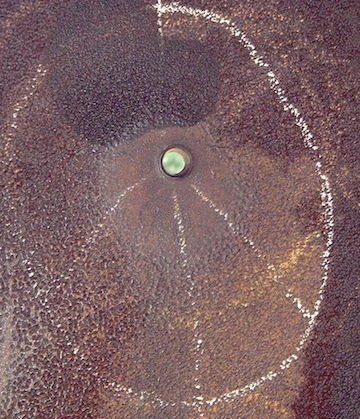# Pie is EverywhereA bullet is formed by revolving the area bounded by the the curve $y = \ln(x)$ from $x = 1$ to $x = e$ about the $x$-axis.

It is then shot straight into a very thick wall (i.e. it does not pierce through the other side at all) making a closed cylindrical hole until it stops moving. Then the bullet is carefully extracted without affecting the hole at all, leaving an empty hole with a pointy end where the bullet once was.

The length of the entire hole is $e+1$. If the volume of the hole can be expressed as $\pi i e,$ where $i$ is a constant, find the value of $i$.

×